# Convert given Binary Search Tree to a Smaller Sum Tree

In this article, we are going to see how to convert a given Binary search tree to a smaller sum tree?
Submitted by Radib Kar, on October 13, 2020

Prerequisite: Convert given binary tree to a greater sum tree

In the previous article, we discussed on converting a binary search tree to a greater sum tree. Tn this article, we are going to see how we can convert a binary search tree into a smaller sum tree which is pretty much reverse of what we did earlier. Still, before discussing the solution, let’s understand what does mean by a smaller sum tree. A Smaller sum tree means the root will have value the same as the sum of the nodes having value less than the root. Let's discuss that with an example.

Below is the binary tree to be considered: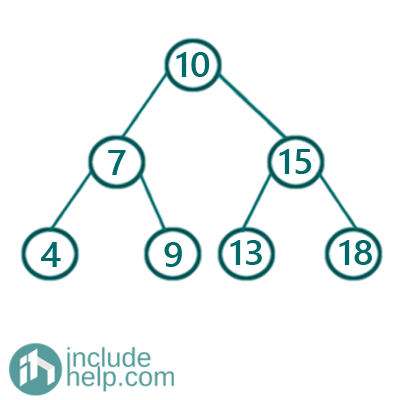For example, the leftmost node 4 doesn't have any value less than this, so that will be replaced by 0. On the other has node 9 has nodes 4 & 7 less than that, so that will be replaced by 4+7=11. If we continue this way the resulting subtree will be: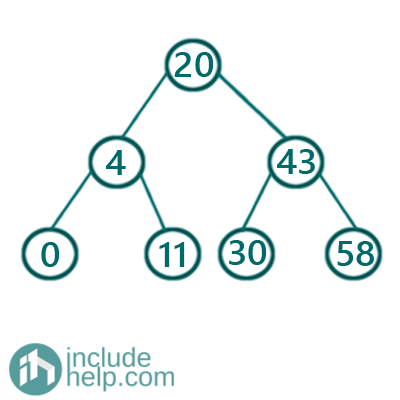Solution:

Of course, there is a naïve way, where for each node we can find which nodes are smaller than this and can replace the node value with the sum of the less valued nodes. That will take O(n^2) time complexity and will be similar for the binary tree as well. But we can have a better one pass solution using the properties of the binary search tree as we showed in our previous article to convert into a greater sum tree. Here, we need to visit the nodes in sorted order(ascending) and thus we need inorder traversal. And we can use inorder traversal to solve. The algorithm is like below:

1. Have a variable sum which will store sum while the inorder traversal. Initiate sum to 0
2. Do inorder traversal like below:
1. ```// Base case
if the root is NULL
return;
```
2. Recur for the left subtree first as it's a normal inorder traversal
3. store the current sum to variable previous sum and update sum as sum+ root value
change the root value as previous sum which is the sum of the nodes already traversed and those are surely less than the node since they fall in the left subtree only
4. Recur for the right subtree now

Below is the implementation of the above algorithm

```#include <bits/stdc++.h>
using namespace std;

// tree node is defined
class TreeNode {
public:
int val;
TreeNode* left;
TreeNode* right;
TreeNode(int data)
{
val = data;
left = NULL;
right = NULL;
}
};

//inorder traversal for tree
void inorder(TreeNode* root)
{
if (!root)
return;
inorder(root->left);
cout << root->val << " ";
inorder(root->right);
}

// Recursive function to transform a BST to smaller sum tree.
// do a inorder traversal for the BST
void transformToSmallerSumTreeRecur(TreeNode* root, int& sum)
{
// Base case
if (!root)
return;

// Recur for left subtree first since it's normal inorder traversal
transformToSmallerSumTreeRecur(root->left, sum);

// store the previous sum and update sum
int prevSum = sum;
sum = sum + root->val;

// Store old sum in the current node which is sum of the left
// subtree nodes(nodes lesser than the root)
root->val = prevSum;

// Recur for right subtree now
transformToSmallerSumTreeRecur(root->right, sum);
}

void transformToSmallerSumTree(TreeNode* root)
{
//initially sum is 0
int sum = 0;
transformToSmallerSumTreeRecur(root, sum);
}

int main()
{
//Tree built as per example
TreeNode* root = new TreeNode(10);
root->left = new TreeNode(7);
root->right = new TreeNode(15);
root->left->left = new TreeNode(4);
root->left->right = new TreeNode(9);
root->right->left = new TreeNode(13);
root->right->right = new TreeNode(18);

cout << "Inorder Traversal of the given tree\n";
inorder(root);
cout << "\nconverting to smaller Sum tree\n";
transformToSmallerSumTree(root);
cout << "Conversion completed\n";
cout << "Inorder Traversal of transformed smaller sum tree:\n";
inorder(root);

return 0;
}
```

Output:

```Inorder Traversal of the given tree
4 7 9 10 13 15 18
converting to smaller Sum tree
Conversion completed
Inorder Traversal of transformed smaller sum tree:
0 4 11 20 30 43 58
```

Dry run

Below is the step by step dry run of the above implementation. Since it's a reverse inorder traversal, it will first reach the rightmost node.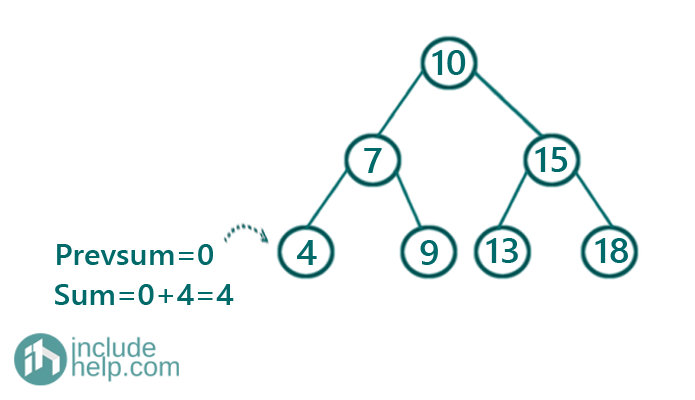Initially, sum is 0 so that will be transferred to the leftmost node via prevsum and the sum updated as sum+root->val=0+4=4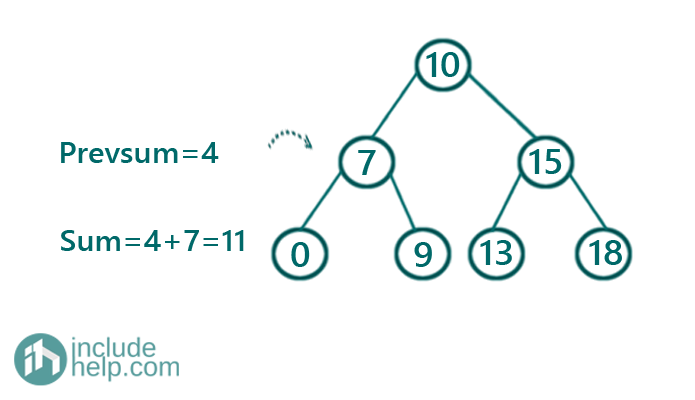Now, sum is 4 so that will be transferred to the current node via prevsum and the sum updated as sum+root->val=4+7=11

You can check that 4 is the only smaller node than 7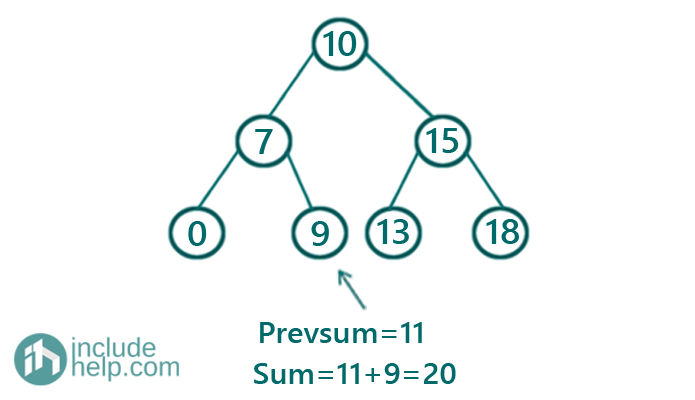Now,  sum is 11 so that will be transferred to the current node via prevsum and the sum updated as sum+root->val=11+9=20

You can check that 4 & 7(total 11) are the only smaller node than 9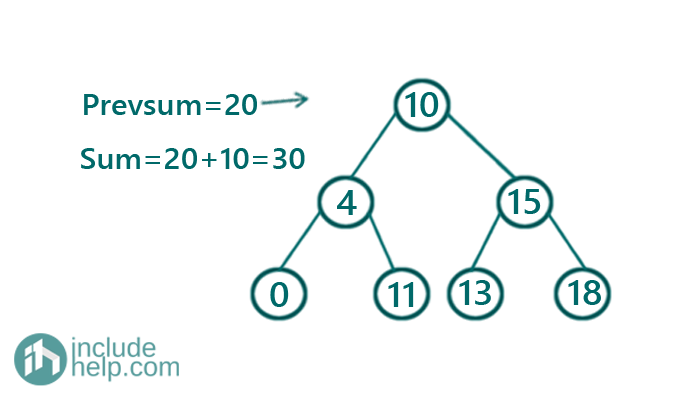Now, sum is 20 so that will be transferred to the current node via prevsum and the sum updated as sum+root->val=20+10=30

You can check that 4, 7, 9(total 20) are the smaller nodes than 10

Similarly,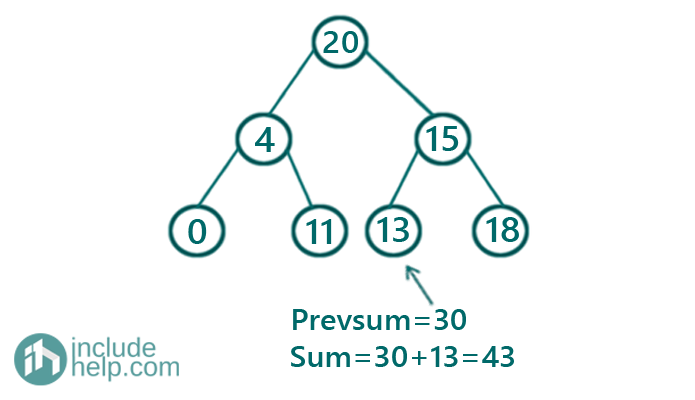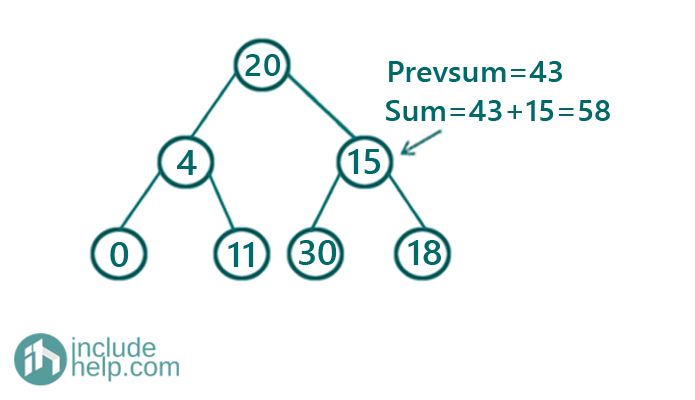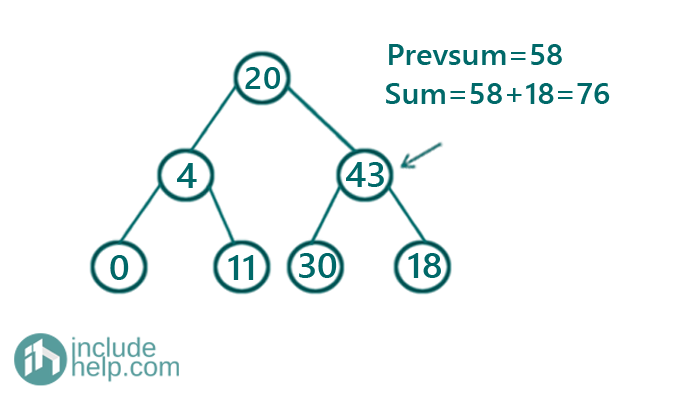Finally, the smaller sum tree is: